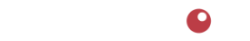# Breakeven Units Calculator

This calculator determines the number of units of a product that must be sold during a period to cover expenses and avoid a loss duing that period.

Breakeven Units is defined as Expenses Per Period divided by Gross Profit Per Unit (also called "Contribution Margin Per Unit").
Contribution Margin Per Unit is defined as Selling Price minus Unit Cost.

To use this calculator, enter the unit cost and unit selling price of the food product. Enter the expenses for the period being analyzed. Then press the [Calculate] button.
The calculated Breakeven Units will appear in the space labeled "Breakeven Units".
The number of units is rounded up to the next highest whole number.

Be sure to check out all our calculators located here.

### Breakeven Units Calculator

Explanation:

Be sure to check out all our calculators located here.4110 SE Hawthorne Blvd. #739

Portland OR 97214

503.234.7199

Notice | Privacy